# Increasing the current handling capability of linear voltage regulators

#### khatus

Joined Jul 2, 2018
93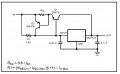I want to know how the above equation arrives.I Have tried but I can't make it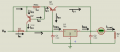For transistor Q2,
VBE(Q2) = 0.8 = ISC × RSC
From the output side of the regulator we get,
From the input side of the regulator we get,
IREG = IB1 +IC2 +IR1
IB2 =( β+1)× IE2

#### WBahn

Joined Mar 31, 2012
27,880
Think about how the circuit behaves as output current is drawn, starting from no output current, as the current draw is increased a little bit, what is the path of that current through the external components? Are the transistors on or off? What is happening to the Vbe of the transistors while this happens? At what point does one of the transistors turn on? What is the path of the current then? What happens as even more output current is drawn? At what point does the other transistor turn on? What happens as the output current attempts to increase even more?

Also, note that the equation for R1 that you give is not correct, at least if the normal order of operations is used. What you have is

$$R1 \; = \; \frac{ \beta V_{BE(Q_1)}} {I_{REG\;Max}} \left( \beta + 1 \right) \; - \; I_{O\;Max}$$

Which isn't even dimensionally consistent.

•#### khatus

Joined Jul 2, 2018
93
Can you show me the derivation?

#### MisterBill2

Joined Jan 23, 2018
13,778
Some versions of that circuit show a diode in series with R1 to provide Vbe compensation, without ever explaining why such would be needed. To provide overload protection for the pass transistor the solution,for R1 is that the current through the pass transistor reaches it's maximum at the same time the regulator reaches it's overcurrent limiting point. Because both resistor current and base current both flow into the regulator, and because Vbe of the transistor varies a bit with the current, it gets a bit complicated, hence some putting that diode in series with R1. That keeps the two voltage drops closer to dependent on the current. So at that point R1 sets the ratio of regulator current to transistor collector current. So that is how R1 is calculated.
But most users simply pick a small value of 5 ohms or 4.7 ohms, depending on what is convenient.

#### dl324

Joined Mar 30, 2015
15,453
Can you show me the derivation?
The equation shown can't be derived because it's wrong. Sadly, other manufacturers have the same incorrect equation.

If you look at the two circuits/equations I posted, you'll notice that short circuit protection is ignored.

If you use the circuit in Fig. 24, define Ir to be the current through R1, label the current out of the base of Q1 as Ib, label Iq1 as βIb, and ignore the quiescent current from the regulator, you'd have: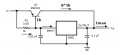From that, it follows that:
$$\large I_{R}=I_{max}-(\beta +1)I_B$$
and
$$\large R1 = \frac{V_{BE(Q1)}}{I_{R}}=\frac{0.9V}{I_{max}-(\beta +1)I_B}$$

This is a better way to divide current between the regulator and pass transistor from National Semiconductor: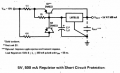This circuit has the regulator operating at its maximum current rating when the load is drawing the maximum current. The regulator self-protection circuitry will protect the external pass transistor; assuming you provide sufficient heat sinking.

Last edited:
•#### WBahn

Joined Mar 31, 2012
27,880
Can you show me the derivation?
First off, I disagree with their equation, although it is in the ballpark from a design standpoint.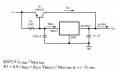One thing to keep in mind is that the current polarities for a PNP are reversed relative to an NPN, so Ic, Ie, and Ib are all negative, as is Vbe and Vce.

So, for instance, Ic for Q1 is related to their I_Q1 by

I_Q1 = -Ic

I plan to use the transistor voltages and currents properly, but when I talk about a voltage or a current increasing, I'm going to be talking about an increase in magnitude, not arithmetic value.

We'll ignore the current consumed by the regulator itself, which flows from the INPUT to GND. This is a reasonable assumption since it is typically less than 10 mA compared to the 2+ A output capability).

So what happens when just a little bit of current is being drawn by the load?

Well, the only way that it can come from Q1 is if the base voltage is low enough to start turning the transistor on. But that requires a sufficient drop across R1 to generate the needed Vbe voltage and any current flowing in R1 is flowing into the regulator and out to the load. So the first chunk of current flows is supplied by the regulator and not the bypass circuit.

How much is this first chunk? Well, the transistor will start turning on when Vbe gets larger than some threshold. The data sheet chose 0.9 V (making Vbe = -0.9 V). If we look at the 2N6133 datasheet, I don't know how reasonable this is. This is a pretty obsolete part and the three versions of the data sheet I tracked down don't give typical Vbe values, just a max value of -1.4 V at an Ic of -2 A and a Vce of -4 V. But, for argument's sake, let's accept TI's value of 0.9 V.

So that means that the initial load current, I_ini, comes through R1 and the regulator.

I_ini = -Vbe / R1 = 0.9 V / R1

As the load tries to pull more current, if more current flows through R1, then the Vbe of Q1 increases, which turns it on and let's current bypass the regulator. Hence, the voltage across R1 becomes pretty firmly clamped by Q1 and remains fairly close to 0.9 V, so we can reasonably assume that the current in R1 never rises appreciable above I_ini. A rule of thumb with silicon PN junctions (at room temperature) is that the voltage increases by 60 mV for every increase in current of a factor of 10. The 2N6133 has an absolute collector current of -7 A, If we assume that the Vbe of -0.9 V is for a collector current of just -7 mA, that means that at -7 A, the Vbe would have increased to -1.08 V and the current through R1 would increase by about 20%. So we are not being too outlandish by assuming that it clamps at I_ini.

The regulator input current, I_reg, does increase a bit, namely by the Q1 base current. But this is reasonably small compared to the bypass current. The 2N6133 has a beta that can range from 20 to 100, so I_reg increases by between 1% and 5% of the bypass current.

If the bypass current is equal to I_Q1, then the base current in Q1 is -I_Q1/ß, Thus the total regulator input current is

I_reg = (-Vbe / R1) + (I_Q1/ß)

The maximum current that we want to allow through the regulator is I_regmax (we could use the datasheet value of 1.5 A, but it's better to use some fraction of it) is

I_regmax = (-Vbe / R1) + (I_Q1max/ß)

This let's us determine the maximum transistor bypass current we want to allow.

I_Q1max = ß[(Vbe / R1) + I_regmax]

The total output current, Io, is

Io = I_reg + I_Q1 = (-Vbe / R1) + (I_Q1/ß) + I_Q1
Io = (-Vbe / R1) + (I_Q1/ß) + I_Q1
Io = (-Vbe / R1) + ((ß+1)/ß)(I_Q1)

If we pick our design's maximum total output current to be Io_max, then we have:

Io_max = (-Vbe / R1) + ((ß+1)/ß)(I_Q1max)
Io_max = (-Vbe / R1) + ((ß+1)/ß)(ß[(Vbe / R1) + I_regmax])
Io_max = (-Vbe / R1) + (ß+1)[(Vbe / R1) + I_regmax]
Io_max = (-Vbe / R1) + (ß+1)(Vbe / R1) + (ß+1)·I_regmax
Io_max = ß·(Vbe / R1) + (ß+1)·I_regmax

This last equation might seem odd at first glance. So let's look at it from a different perspective.

Io_max = I_regmax + I_Q1max

I_Q1max is ß times the maximum base current and the maximum base current is I_regmax minus I_ini

We'll define I_Q1b to be the current flowing down out of the base of Q1 (so it is the negative of the base current, Ib)

Io_max = I_regmax + ß·I_Q1bmax

I_Q1bmax = I_regmax - I_ini
I_Q1bmax = I_regmax + (Vbe / R1)

Io_max = I_regmax + ß·[I_regmax + (Vbe / R1)]
Io_max = ß·(Vbe / R1) + (ß+1)·I_regmax

So the same result as above. Remember that Vbe is negative, so this is really saying that we have the max regulator current + the bypass current as if all of the regulator current came through the base, then minus the collector current we don't get because I_ini does NOT go through the base.

Now we solve for R1:

ß·(Vbe / R1) = Io_max - (ß+1)·I_regmax

R1 = (ß·Vbe) / (Io_max - (ß+1)·I_regmax)

If we take into account that their Vbe is the negative of ours, we have

R1 = (ß·(-Vbe)) / [(ß+1)·I_regmax - Io_max] <==============

This, then, is their equation for R1 if you set -Vbe = 0.9 V

Now let's massage this a bit by dividing numerator and denominator by ß on the right-hand-side.

R1 = (-Vbe) / [(1+1/ß)·I_regmax - Io_max/ß]

Now note what they say about ß, namely that

ß >= Io_max / I_regmax

This requirement is to ensure that R1 doesn't come out to be negative.

Now recall that

R1 = (-Vbe) / I_ini

This means that I_ini can be expressed as

I_ini = (1+1/ß)·I_regmax - Io_max/ß
I_ini = (ß+1)·I_regmax - Io_max

This result should now make sense.

One thing to note is that their equation starts off

R1 = 0.9 V / I_reg

This is NOT correct. First off, I_reg is a variable that ranges from 0 A to I_regmax. Second, I_reg, as they have defined it, does not all flow through R1. Some of it comes from the base of Q1. Fortunately, they didn't carry that mistake through their work.

•khatus